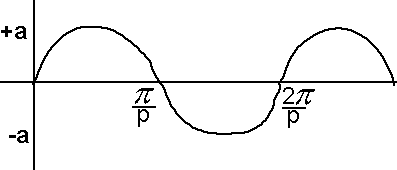# Maths - Trigonometry

## Periodic functions of the type y = a sin px

The graph of y = a sin(px) is similar to that of the sin graph of y = sin(x). Instead of varying or oscillating between +1 and -1, a sin(px) will vary between +a and -a. 'a' is called the amplitude and affects only the scale for y. The graph will repeat itself when the angle px increases by 360° or 2π radians. i.e. when x increases by 360°/p or 2π/p radians thus the period is 2π/p radians.Thus 5 sin(2x) has a period of 2π/2 = π radians and amplitude 5. Hence there are 2 waves between 0° and 360°.

### Example

Sketch the curve for y = 3 sin(x+60°)y = 3 sin(x+60°) is said to have a phase lead of 60° since its values are those of y = 3 sin(x) with x increased by 60°. y = 3 sin(x-60°) is said to lag by 60°.

## Solving Trig Equations

Here are some suggestions for solving trig identities:

1. Change secant, cosecant and cotan into cos, sin and tan.
2. rewrite in terms of sin and cos (use tan = sin/cos)
3. If in second degree (ie cos2 or sin2) look for posible cos2A + sin2A = 1 relationship.
4. If it contains cos2 or sin2 change sin2 into cos2-1 and solve resulting quadratic.
5. If all this fails convert to an infinite series.
6. If this fails plot as a graph.

## Plotting trig functions

### Example 1

y = 3 sin(2x) + sin x### Example 2

y = 3 sin(x/2) - cos xmetadata block see also: Correspondence about this page Book Shop - Further reading. Where I can, I have put links to Amazon for books that are relevant to the subject, click on the appropriate country flag to get more details of the book or to buy it from them.Mathematics for 3D game Programming - Includes introduction to Vectors, Matrices, Transforms and Trigonometry. (But no euler angles or quaternions). Also includes ray tracing and some linear & rotational physics also collision detection (but not collision response). Other Math Books

This site may have errors. Don't use for critical systems.